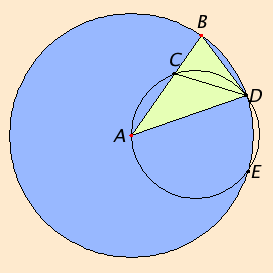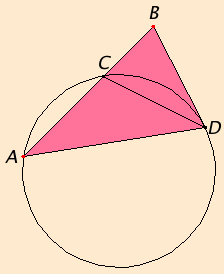# Proposition 10

To construct an isosceles triangle having each of the angles at the base double the remaining one.

Set out any straight line AB, and cut it at the point C so that the rectangle AB by BC equals the square on CA. Describe the circle BDE with center A and radius AB. Fit in the circle BDE the straight line BD equal to the straight line AC which is not greater than the diameter of the circle BDE.

IV.5

Join AD and DC, and circumscribe the circle ACD about the triangle ACD.Then, since the rectangle AB by BC equals the square on AC, and AC equals BD, therefore the rectangle AB by BC equals the square on BD.

III.37

And, since a point B was taken outside the circle ACD, and from B the two straight lines BA and BD fall on the circle ACD, and one of them cuts it while the other falls on it, and the rectangle AB by BC equals the square on BD, therefore BD touches the circle ACD.

III.32

Since, then, BD touches it, and DC is drawn across from the point of contact at D, therefore the angle BDC equals the angle DAC in the alternate segment of the circle.

Since, then, the angle BDC equals the angle DAC, add the angle CDA to each, therefore the whole angle BDA equals the sum of the two angles CDA and DAC.

I.32

But the exterior angle BCD equals the sum of the angles CDA and DAC, therefore the angle BDA also equals the angle BCD.

I.5

But the angle BDA equals the angle CBD, since the side AD also equals AB, so that the angle DBA also equals the angle BCD.

Therefore the three angles BDA, DBA, and BCD equal one another.

I.6

And, since the angle DBC equals the angle BCD, the side BD also equals the side DC.

I.5

But BD equals CA by hypothesis, therefore CA also equals CD, so that the angle CDA also equals the angle DAC. Therefore the sum of the angles CDA and DAC is double the angle DAC.

And the angle BCD equals the sum of the angles CDA and DAC, therefore the angle BCD is also double the angle CAD.

But the angle BCD equals each of the angles BDA and DBA, therefore each of the angles BDA and DBA is also double the angle DAB.

Therefore the isosceles triangle ABD has been constructed having each of the angles at the base DB double the remaining one.

Q.E.F.

## Guide

The goal of the proposition is to construct a 36°-72°-72° isosceles triangle ABD. It’s actually constructed on a given side AB. The base will equal the larger part of AB when AB is cut at a point C so that AB BC = AC2. The constuction for that cut was given in proposition II.11. The difficulty of the proof is showing that this construction results in the desired triangle. Cutting AB at that point is also called cutting the line “in extreme and mean ratio,” see VI.Def.3 for the definition of “extreme and mean ratio,” and see proposition VI.30 for details.

 Euclid uses a surprising amount of the theory of circles from Book III. After drawing circle ACD, he uses III.37 to conclude from AB BC = DB2 that the line DB is tangent to the circle. Next, he uses III.32 to conclude that the angle BDC between the tangent DB and the chord DC equals the angle CAD which cuts off that chord. At this point Euclid has shown that one of the two angles at D, namely angle BDC, equals the angle A. When he shows that the other, namely angle CDA also equals angle A, then since the triangle ABD is isosceles, he will have shown each of the base angles of triangle ABD is twice the vertex angle A, and the proof will be complete.The rest is relatively easy. First, the small triangle BCD is isosceles, a fact that can be seen from the following equation about angles:

B = BDA = BDC + CDA = CAD + CDA = BCD.

Therefore, the sides CD and BD are equal, but from the original construction, BD = CA. Hence, the triangle ADC is also isosceles, so the two angles CDA and A are equal, as needed.

Euclid could have split the statement and the proof of this proposition into two. The first part would state that if an isosceles triangle has its base equal to a segment of its side so that square on the base equals the rectangle contained by the side and the remaining segment of the side, then each base angle of the triangle is twice the vertex angle. Most of the proof of this proposition IV.10 is actually a proof of this first part. The other part would be the constuction.

There is a converse of this proposition, one the Euclid did not state. Namely, if an isosceles triangle has each base angle equal to twice the vertex angle, then the base is equal to a segment of its side so that square on the base equals the rectangle contained by the side and the remaining segment of the side. In other words, 36°-72°-72° isosceles triangles are characterized by this property.

The triangle ABD constructed in this proposition is one of ten sectors of a regular decagon (10-gon). Thus, it is one short step from this proposition to the construction of a regular decagon inscribed in a circle. If alternate vertices of a regular decagon are connected, then a regular pentagon is formed which is inscribed in the circle. It is unclear why Euclid did not use such a construction rather than the one he chose in the next proposition

#### An alternate proof involving similar triangles

It was probably Euclid who made a concerted effort to include as many propositions that he could in the first four books that did not rely on proportions. The theory of similar triangles is not broached until Book VI which depends on the theory of proportion in Book V. The clever proof that Euclid gave to this proposition does not depend on similar triangles, and so it could be placed here in Book IV. There is, however, a simpler proof that does depend on similar triangles.

As Euclid does, begin by cutting a straight line AB at the point C so that the rectangle AB by BC equals the square on CA (II.11). Otherwise said, the straight line AB has been cut in extreme and mean ratio at C so that the proportion AB : AC = AC : BC holds. (See VI.Def.3, VI.17, and VI.30.) Next, construct an isosceles triangle with one side AB, a second side AD equal to side AB, and the base equal BD equal to AC (I.22). Then we have the proportion AD : BD = BD : BC. Therefore, two triangles ADB and DBC have one angle equal to one angle (angle D of the first triangle equals angle B of the second) and the sides about the equal angles proportional. Therefore, by VI.6, the triangles are equiangular. It easily follows that both triangles have their base angles each equal to twice their vertex angles.

#### Use of this proposition

This construction was designed to be used in the next proposition which inscribes a regular pentagon in a circle.